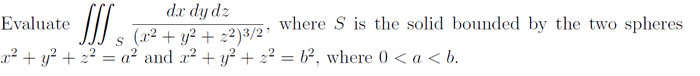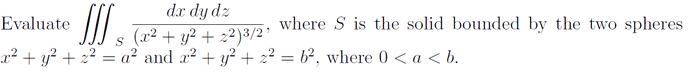# Triple integral spherical coordinates.

## Homework Statement

Here is the question given:## The Attempt at a Solution

So i set p as x^2 + y^2 + z^2

so p lies in between b and a.

But how do i find the restrictions on the two angles, theta and phi?

LCKurtz
Homework Helper
Gold Member

## Homework Statement

Here is the question given:## The Attempt at a Solution

So i set p as x^2 + y^2 + z^2

so p lies in between b and a.

But how do i find the restrictions on the two angles, theta and phi?

Hopefully you mean $\rho=\sqrt{x^2+y^2+z^2}$. Draw a picture. What do $\phi\hbox{ and }\theta$ usually range through for spheres?